### IMO Shortlist 2003 problem G1

Kvaliteta:
Avg: 3,0
Težina:
Avg: 6,0
Let$ABCD$ be a cyclic quadrilateral. Let$P$,$Q$,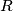$R$ be the feet of the perpendiculars from$D$ to the lines$BC$,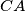$CA$,$AB$, respectively. Show that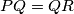$PQ=QR$ if and only if the bisectors of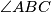$\angle ABC$ and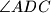$\angle ADC$ are concurrent with$AC$.
Izvor: Međunarodna matematička olimpijada, shortlist 2003